(1) The calculation of the outmost layer

1) Velocity redshift

When the outmost layer was expanded from  a0   to  a ,  to the theorem of kinetic energy we have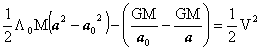from this follows(14)

or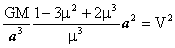therefore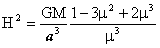(15)

thus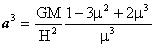(16)

using formula (11)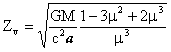(17)

2)  Repulsion  redshift

A photon is shot at the center in the universe. It follows from formula (3) that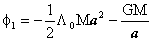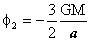substituting the expression for the  f1  and  f2  in formula (12) , We obtain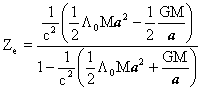(18)

or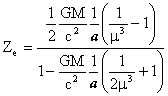(19)

3)  Method of computation

We properly choose μ, M  and  H , and than compute  a ,  but the a must satisfy the relation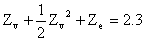(20)

Thus we have finded μ, M, H  and  a  (formulae 16, 17 and 19).